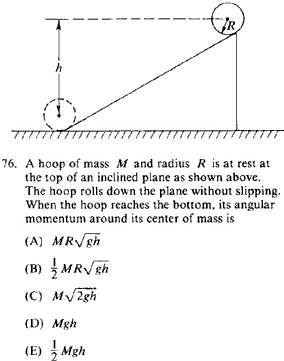GR 8677927796770177 | # Login | Register

GR8677 #76
Problem
 GREPhysics.NET Official Solution Alternate SolutionsMechanics}Conservation of Energy

Recall the formula for inertia . A hoop has constant , thus the integral is trivial and . One can break up the kinetic energy into the pure rolling (about center of mass) and pure translation bit,

where one recalls that .

Plug the value of angular velocity into the equation for angular momentum , to get , as in choice (A).Alternate Solutions
 There are no Alternate Solutions for this problem. Be the first to post one!
mvgnzls
2011-09-26 20:24:02
energy before = energy after
Mgh= M + I w=v/r
Mgh= M + (M)
Mgh= M + M
Mgh= M
v=
now, L= R x Mv = RMv = RMsignminus
2010-08-02 14:25:18
Another POE technique here is to realize that the hoop's angular momentum must depend on its radius (think about a hoop with really huge radius versus one with a tiny radius), so only answers (A) and (B) remain. Of course, something more is needed to get the exact answer, but in a pinch you'll be down to a 50-50 random guess at the worst.
 ali82011-06-30 09:44:29 And if you, in general, are unlucky, like me, then it's really useless.
 mpdude82012-04-19 16:05:57 POE is never useless, and if you can narrow it down to a 1 in 2 guess, guessing is a better option than skipping.
 danielsw986672019-09-13 12:56:02 The answer is A. cash loans south africa no credit checkben
2006-07-19 16:23:40
also, units rule out choices (C), (D), and (E). only (A) and (B) have units of angular momentum
 jmason862009-08-10 20:34:14 Totally agreed. I did this.. but they were really mean in giving you a factor of 2 to distinguish the last choice. Damn ETS. Isn't that the first rule of real physics: Factors of 2 don't matter hahaAndresito
2006-03-19 10:39:52
This solution is confusing. Please specify why the factor of (1/2) disappears when you equate the translational energy with the potential energy.

Thank you
 ben2006-07-20 17:16:01 v=R*omega so the translational kinetic energy is equal to the rotational kinetic energy, and that value is (1/2)*M*R^2*omega^2. so when you add 1/2 plus 1/2 you get 1 making the total kinetic energy M*R^2*omega^2. nothing "disappears" here.
 mvgnzls2011-09-26 20:23:25 energy before = energy after Mgh= M + I w=v/r Mgh= M + (M) Mgh= M + M Mgh= M v= now, L= R x Mv = RMv = RM
 danielsw986672019-10-21 05:55:38 Because the answer is A. semenax reviewLaTeX syntax supported through dollar sign wrappers $, ex.,$\alpha^2_0$produces . type this... to get...$\int_0^\infty\partial\Rightarrow\ddot{x},\dot{x}\sqrt{z}\langle my \rangle\left( abacadabra \right)_{me}\vec{E}\frac{a}{b}\$